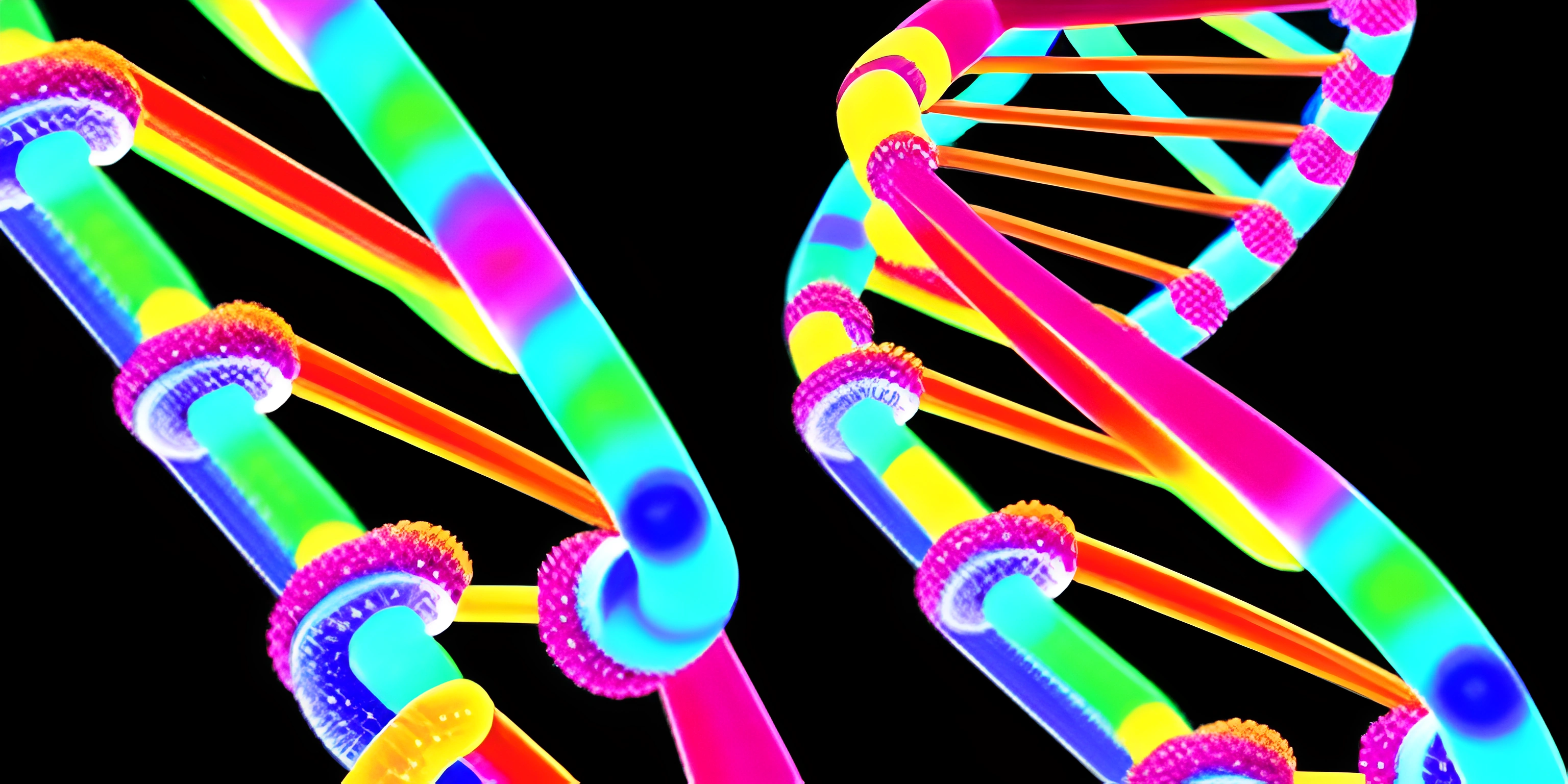# Genetic Algorithms IntroductionNote: this page has been created with the use of AI. Please take caution, and note that the content of this page does not necessarily reflect the opinion of Cratecode.

Welcome to the world of genetic algorithms, where we take inspiration from nature to solve complex problems! Genetic algorithms (GAs) are a type of optimization algorithm that mimic the process of natural selection to find the best solution to a problem. Sit tight, and let's dive into this fascinating approach.

## Foundations

The foundation of genetic algorithms lies in Charles Darwin's theory of evolution, which revolves around the concepts of natural selection and survival of the fittest. In GAs, we create a "population" of possible solutions to our problem, and each "individual" in the population is evaluated based on a predefined "fitness" measure. The fitter individuals have a higher chance of "reproducing" and passing on their characteristics to the next generation.

### Components of a Genetic Algorithm

To understand how genetic algorithms work, let's break down their main components:

1. Population: A collection of potential solutions to the problem, where each solution is referred to as an individual or a chromosome.
2. Fitness function: A measure used to evaluate the performance of each individual in the population.
3. Selection: The process of choosing individuals from the current population to create the next generation.
4. Crossover: Also known as reproduction or recombination, this is the process of combining the traits of two selected individuals to create new offspring.
5. Mutation: The process of introducing small, random changes in the offspring to maintain diversity in the population and avoid premature convergence.

### The Genetic Algorithm Process

With these components in mind, the general process of a genetic algorithm goes as follows:

1. Initialization: Create an initial population of random individuals.
2. Evaluation: Calculate the fitness of each individual in the population.
3. Selection: Choose individuals for reproduction based on their fitness.
4. Crossover: Generate offspring by combining the traits of selected parents.
5. Mutation: Introduce random changes in the offspring.
6. Replacement: Replace the old population with the new offspring.
7. Termination: Repeat steps 2-6 until a stopping criterion is met (such as a maximum number of generations or a satisfactory fitness level).

## Applications of Genetic Algorithms

Genetic algorithms are versatile and can be applied to various optimization problems, including:

1. Traveling Salesman Problem: Finding the shortest route that visits a set of cities and returns to the starting point.
2. Job Scheduling: Allocating tasks to resources to minimize the completion time or cost.
3. Feature Selection: Identifying the most important variables in a dataset for machine learning or data analysis.
4. Game AI: Developing intelligent agents that can adapt and improve their performance in games.
5. Robotics: Optimizing the control parameters and movement strategies for robots.

In conclusion, genetic algorithms are a powerful and flexible optimization technique that can be used to tackle complex problems by mimicking nature's evolutionary process. By understanding their components and process, you can harness the power of GAs to find the best solutions in various domains. Happy evolving!

## FAQ

### What are genetic algorithms and how do they work?

Genetic algorithms are a type of optimization and search technique inspired by the process of natural selection. They work by iteratively generating a population of candidate solutions, evaluating their fitness, and then applying genetic operations such as crossover (mating), mutation, and selection to create a new generation of solutions. Over time, the algorithm converges towards an optimal or near-optimal solution to the problem at hand.

### What are some common applications of genetic algorithms?

Genetic algorithms are versatile and can be applied to a wide range of problems, such as:

• Function optimization
• Machine learning and pattern recognition
• Scheduling and resource allocation
• Game playing and strategy optimization
• Robotics and control systems
• Bioinformatics and gene regulatory network modeling

### How do you evaluate the fitness of a solution in genetic algorithms?

A: Evaluating the fitness of a solution depends on the specific problem being solved. Generally, a fitness function is defined that measures the quality of a candidate solution in the context of the problem. A higher fitness value indicates a better solution. For example, if you're using a genetic algorithm to optimize a function, the fitness function might be the function itself, and the goal would be to find the input that results in the maximum or minimum output value.

### What genetic operations are used in genetic algorithms and what do they do?

A: The main genetic operations used in genetic algorithms are crossover, mutation, and selection:

• Crossover (mating): This operation combines the genetic material of two parent solutions to produce one or more offspring. It mimics the process of recombination in biological reproduction, allowing the offspring to inherit traits from both parents.
• Mutation: This operation introduces small random changes in a solution's genetic material, helping to maintain diversity within the population and prevent premature convergence to suboptimal solutions.
• Selection: This operation determines which solutions will be used as parents for the crossover operation and which solutions will be carried over to the next generation. Typically, solutions with better fitness values have a higher probability of being selected.

### How do you know when to stop a genetic algorithm?

A: Stopping criteria for genetic algorithms may include:

• A predefined number of generations have been completed
• The best fitness value found has reached a certain threshold or target
• The average fitness value of the population has converged, indicating that further improvements are unlikely
• A specific amount of time or computational resources have been exhausted The choice of stopping criteria depends on the specific problem being solved and the desired balance between solution quality and computational efficiency.